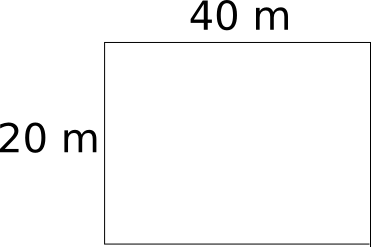Question 22

# Neeraj has agreed to mow a lawn, which is a 20 m × 40 m rectangle. He mows it with 1 m wide strip. If Neeraj starts at one corner and mows around the lawn toward the centre, about how many times would he go round before he has mowed half the lawn? (Round off the answer to two decimal digits)

SolutionTo mow half of lawn is to mow 400 squares of 1m width each. Neeraj mows 40+18+40+18 = 116 squares of 1m width each in 1st round, in 2nd round he mows 38+16+38+16 = 108 squares and in 3rd round, he mows 36+14+36+14 = 100 squares. So, in total, he mows 324 squares; but he needs 400-324 = 76 more.

If he covers the 4th round completely, he mows 92 squares, to cover only 76, he needs to cover 76/92 = 0.826 round.

So in total 3.826 $$\approx$$ 3.83 rounds are required.